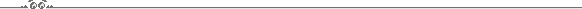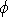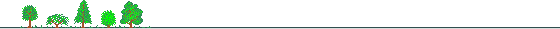Wise Home | Utilities

## Horizontal CoordinatesA coordinate system based on the local horizon. The coordinates lines of this system are the:
Azimuth (Az) - measured along the horizon from the North eastwerd. (e.g. 90o - is East, 180o is South etc.)
Altitude (Alt) (or Elevation, or zenith distance=90o-Altitude) - This is the angle between the object and the horizon measured on the great circle defined by the zenith and the object.
The Transformation between the horizonatl coordinates system and the equatorial coordinates system is given by:
 cos(Alt) sin(Az) = -cossin h cos(Alt) cos(Az) = sincos- coscos h sinsin(Alt) = sinsin+ coscos h cosWhereis the Declination, h is the Hour Angle andis the geocentric latitude. (These formule, don't take into account the refraction in the Earth atmosphere.

## Convert Equatorial Coordinates to Azimuth and Altitude

(without refraction corrections)
 North Latitude o R.A. h Dec. o L.S.T. h

 Azimuth o Altitude oConstructed: November 1998, by: Eran Ofek